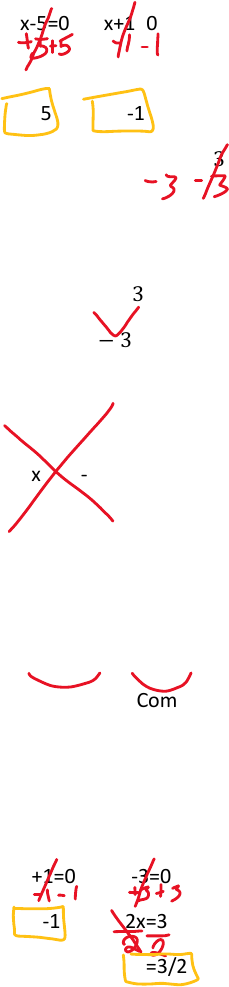Class Notes (1,100,000)
US (490,000)
WSU (30)
MAT (8)
Lecture 1

# MATH 111 Lecture Notes - Lecture 1: Imaginary Number, Quadratic Formula

This preview shows page 1. to view the full 4 pages of the document.8/27/2018
1. Zero-Product Property (use every time we factor)
If    then a=0 or b=0
Ex. Solve-
(x-5)(x+1)=0
x-5=0 or x+1=0
x=5 x=-1
ex. Solve-  
2x^2-x-3=0 Standard form- everything on one side and 0 on the other
(2x-3)(x+1)=0 Using the guess and check method
     If you get the opposite, switch the signs of the numbers. If you get a
  completely wrong number, switch which parentheses the numbers are in.
Product Using the AC method
-6 2x times -3x should be -6 and 2x+-3x should be -1
2x -3x A=2, B=-1, C=-3
-1
Sum^
      (factor)
Common Common
Factor Factor
2x(x+1) -3(x+1) =0
(x+1)(2x+3)
X+1=0 2x-3=0
X=-1 2x=3
X=3/2